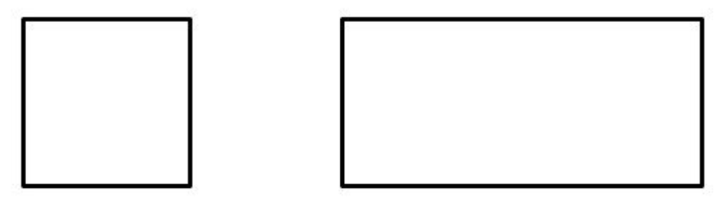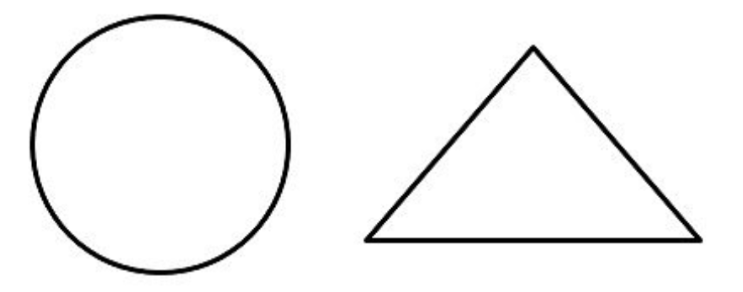QUESTION

# Give two examples of non-similar figures.

Hint: Two figures that have the same shape are said to be similar. When two figures are similar, the ratios of the lengths of their corresponding sides are equal. Similarly, two figures are said to be non-similar if either they are not similar or if they do not have matching properties.

Complete Step-by-Step solution:
To proceed this question, we will first of all define what are Similar figures and what are non-similar figures.
Two figures that have the same shape are said to be similar. When two figures are similar, the ratios of the lengths of their corresponding sides are equal.
Similarly defining Non-similar figures, we have,
Two figures are said to be non-similar if either they are not similar or if they do not have matching properties.
We will try to give the most basic examples of non-similar figures.
(1) The first example is of a square and a rectangle. They are non-similar figures and hence do not have any similarity in their properties.
They can be drawn as below,(2) Another most common example of non-similar figures are two very different figures Circle and a triangle. One of them has sharp ends and the other having no ends at all. They differ in all the properties wrt each other and hence come in the category of non-similar figures. They can be shown as below,Hence, we obtain two examples of non-similar figures.

Note: The possibility of error in this question can be getting confused in between the figures such as square and rectangle. Although they may have properties in similar looks but they come under the category of non-similar figures because of their different diagonal properties.